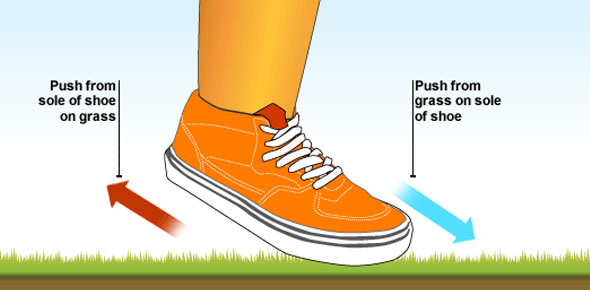# Motion & Forces - Chapter 3

16 Questions | Attempts: 169Settings• 1.
The force that attracts two masses to each other is called
• A.

Air resistance

• B.

Friction

• C.

Gravity

• D.

Pressure

• 2.
A hollow plastic ball and a solid metal ball are dropped from the same height in a vacuum.  With no air resistance, gravity is the only force acting on the balls.  What do you predict will happen?
• A.

The balls will fall at the same rate

• B.

The metal ball will fall faster

• C.

The plastic ball will fall faster

• D.

The larger ball will fall faster

• 3.
After a space shuttle is launched into orbit, gravity
• A.

Gives it a speed greater than 11,000 m/s

• B.

No longer affects the astronauts on board

• C.

Makes it impossible for it to stay in orbit for a long period of time

• D.

Continues to pull it toward the center of Earth

• 4.
Michelle is sliding a box across the floor.  She feels the box resisting her push.  The resistance is caused by
• A.

Pressure

• B.

Friction

• C.

Gravity

• D.

Motion

• 5.
Mark wants to slide a chair across a carpeted floor.  He could reduce the effect of friction by
• A.

Placing the chair so that a smaller area is in contact with the floor

• B.

Putting a plastic sheet on the floor before he slides the chair

• C.

Asking a friend to sit in the chair while he pushes it

• D.

Increasing the force with which he pushes the chair

• 6.
Roger is designing a new model rocket.  He wants it to have as little air resistance as possible.  What should he do?
• A.

Decrease its surface area

• B.

Lower its temperature

• C.

Increase its speed

• D.

Make its surface rougher

• 7.
To find pressure, you
• A.

Multiply the mass by the gravitational constant g

• B.

Divide the force by the area over which it is exerted

• C.

Divide the total mass by the volume

• D.

Multiply the mass by the acceleration

• 8.
As Lisa climbs a mountain, the air pressure relative to sea level
• A.

Increases; there is less air below her than above her

• B.

Stays the same no matter how high she climbs

• C.

Decreases; there is more air below her than above her

• D.

Changes depending on how fast Lisa climbs

• 9.
The water in a fast-moving stream exerts less pressure on the bottom than the water in a slow moving stream.  This is an example of
• A.

Pascal's principle

• B.

Buoyancy

• C.

Density

• D.

Bernoulli's principle

• 10.
Naomi squeezes the wide end of a tube of toothpaste.  The pressure transmitted to the opposite end of the tube is
• A.

Equal to the pressure Naomi exerts

• B.

Less to the pressure Naomi exerts

• C.

Greater to the pressure Naomi exerts

• D.

Dependent on the area of the opposite end of the tube

• 11.
What unit is used to measure pressure?
• A.

N (Newton)

• B.

Pa (Pascal)

• C.

Kg (kilogram)

• D.

M (meter)

• 12.
What pressure is exerted when a force of 50N is applied to a box with and area of 4 meters squared?  The formula for pressure is P=F/A
• A.

0 Pa

• B.

2 Pa

• C.

25 Pa

• D.

12.5 Pa

• 13.
Box A (Area = 4 square meters)Box B (Area = 0.75 square meters)Which box exerts the greatest pressure if they have the same area on the floor.
• A.

Box A

• B.

Box B

• C.

Option 3

• D.

Option 4

• 14.
You are going to drop two balls from the same height.  They both weigh 50 grams.  One ball is the size of a basketball and the other ball is the size of a tennis ball.  Predict which ball will hit the ground first.
• A.

The tennis ball will hit the ground first because it has a smaller surface are so it has less air resistance

• B.

They will both go upward instead of fall to the ground

• C.

The larger ball will hit the ground first because it has more surface area and has more air resistance

• D.

Option 4

• 15.
On land, Melanie cannot lift her little brother.  In the water, though, she can lift him easily.  What force helps her in the water?
• A.

Frictional force

• B.

Gravitational force

• C.

Buoyant force

• D.

Option 4

• 16.
Robin is pushing an empty cart across the floor at a steady speed.  Is Robin fills the cart with rocks, predict how her force on the cart will compare with her force on the empty cart if she pushes with the same steady speed?
• A.

Robin's force needs to be smaller to push the more massive cart

• B.

Robin's force needs to be greater to push the more massive cart

• C.

Robin's force needs to be the same to push the more massive cart

• D.

It really doesn't matter how the mass of the cart changes

## Related TopicsBack to top
×

Wait!
Here's an interesting quiz for you.